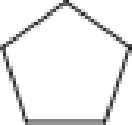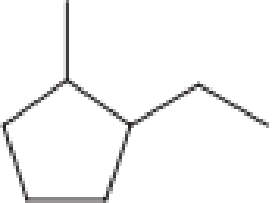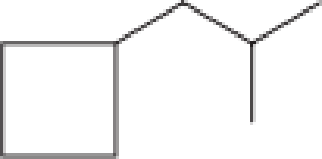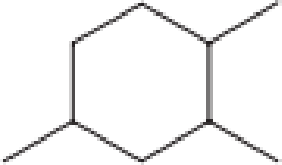Chapter 1, Problem 1.96EP### Organic And Biological Chemistry

7th Edition
STOKER + 1 other
ISBN: 9781305081079

#### Solutions

Chapter
Section### Organic And Biological Chemistry

7th Edition
STOKER + 1 other
ISBN: 9781305081079
Textbook Problem

# What is the molecular formula for each of the following cycloalkane molecules?a.b.c.d.(a)

Interpretation Introduction

Interpretation:

The molecular formula for the given cycloalkane structure has to be found.

Concept Introduction:

Organic compounds are the important basis of life.  They include gasoline, coal, dyes, and clothing fibers etc.  The compounds that are obtained from living organisms are termed as organic compounds and those obtained from the earth are known as inorganic compounds.  Organic compounds are found in earth also apart from living organisms.  All the organic compounds contain the element carbon.  Urea was synthesized in the laboratory which is an organic compound.

Hydrocarbons are the organic compounds that contain only hydrogen and carbon atoms.  Hydrocarbon derivatives are the one in which the compounds contain hydrogen and carbon atoms along with one or more additional elements.  The additional elements that can be present in hydrocarbon derivatives are oxygen, nitrogen, sulphur, chlorine, bromine etc.

Hydrocarbons are further classified into two categories.  They are saturated hydrocarbons and unsaturated hydrocarbons.  The hydrocarbons that contain single bonds between carbon atoms in the entire molecule is known as saturated hydrocarbon.  The hydrocarbons that contain atleast one double or triple bond between two carbon atoms in the entire molecule is known as unsaturated hydrocarbon.

Cycloalkanes are a class of saturated hydrocarbons that contain a ring of carbon atoms with or without alkyl substituents on it.  The general molecular formula for cycloalkanes is CnH2n.  “n” is the number of carbon atoms present.  Some of the basic examples of cycloalkanes are cyclopropane (C3H6), cyclobutane (C4H8).

In a line-angle structural formula of cycloalkanes, the intersection of two lines represent a methylene group (CH2 group).  When substituents are present in the cycloalkane ring, three- and four-way intersections of lines are also possible.  CH group is present when there is a three-way intersection and a carbon atom alone is present when there is a four-way intersection.

Explanation

Given cycloalkane is,

The above structure is a line-angle structural formula representation.  This structure has a total of five intersections.  Therefore, the total number of carbon atoms present in the given structure is found to be five.

The molecular formula for the given cycloalkane can be found using the general molecular formula.  General molecular formula for cycloalkane is CnH2n.  The total number of carbon atoms in the given cycloalkane is 5.  This means “n” is five.  Substitution this is the general molecular formula as shown below, the molecular formula can be obtained

(b)

Interpretation Introduction

Interpretation:

The molecular formula for the given cycloalkane structure has to be found.

Concept Introduction:

Organic compounds are the important basis of life.  They include gasoline, coal, dyes, and clothing fibers etc.  The compounds that are obtained from living organisms are termed as organic compounds and those obtained from the earth are known as inorganic compounds.  Organic compounds are found in earth also apart from living organisms.  All the organic compounds contain the element carbon.  Urea was synthesized in the laboratory which is an organic compound.

Hydrocarbons are the organic compounds that contain only hydrogen and carbon atoms.  Hydrocarbon derivatives are the one in which the compounds contain hydrogen and carbon atoms along with one or more additional elements.  The additional elements that can be present in hydrocarbon derivatives are oxygen, nitrogen, sulphur, chlorine, bromine etc.

Hydrocarbons are further classified into two categories.  They are saturated hydrocarbons and unsaturated hydrocarbons.  The hydrocarbons that contain single bonds between carbon atoms in the entire molecule is known as saturated hydrocarbon.  The hydrocarbons that contain atleast one double or triple bond between two carbon atoms in the entire molecule is known as unsaturated hydrocarbon.

Cycloalkanes are a class of saturated hydrocarbons that contain a ring of carbon atoms with or without alkyl substituents on it.  The general molecular formula for cycloalkanes is CnH2n.  “n” is the number of carbon atoms present.  Some of the basic examples of cycloalkanes are cyclopropane (C3H6), cyclobutane (C4H8).

In a line-angle structural formula of cycloalkanes, the intersection of two lines represent a methylene group (CH2 group).  When substituents are present in the cycloalkane ring, three- and four-way intersections of lines are also possible.  CH group is present when there is a three-way intersection and a carbon atom alone is present when there is a four-way intersection.

(c)

Interpretation Introduction

Interpretation:

The molecular formula for the given cycloalkane structure has to be found.

Concept Introduction:

Organic compounds are the important basis of life.  They include gasoline, coal, dyes, and clothing fibers etc.  The compounds that are obtained from living organisms are termed as organic compounds and those obtained from the earth are known as inorganic compounds.  Organic compounds are found in earth also apart from living organisms.  All the organic compounds contain the element carbon.  Urea was synthesized in the laboratory which is an organic compound.

Hydrocarbons are the organic compounds that contain only hydrogen and carbon atoms.  Hydrocarbon derivatives are the one in which the compounds contain hydrogen and carbon atoms along with one or more additional elements.  The additional elements that can be present in hydrocarbon derivatives are oxygen, nitrogen, sulphur, chlorine, bromine etc.

Hydrocarbons are further classified into two categories.  They are saturated hydrocarbons and unsaturated hydrocarbons.  The hydrocarbons that contain single bonds between carbon atoms in the entire molecule is known as saturated hydrocarbon.  The hydrocarbons that contain atleast one double or triple bond between two carbon atoms in the entire molecule is known as unsaturated hydrocarbon.

Cycloalkanes are a class of saturated hydrocarbons that contain a ring of carbon atoms with or without alkyl substituents on it.  The general molecular formula for cycloalkanes is CnH2n.  “n” is the number of carbon atoms present.  Some of the basic examples of cycloalkanes are cyclopropane (C3H6), cyclobutane (C4H8).

In a line-angle structural formula of cycloalkanes, the intersection of two lines represent a methylene group (CH2 group).  When substituents are present in the cycloalkane ring, three- and four-way intersections of lines are also possible.  CH group is present when there is a three-way intersection and a carbon atom alone is present when there is a four-way intersection.

(d)

Interpretation Introduction

Interpretation:

The molecular formula for the given cycloalkane structure has to be found.

Concept Introduction:

Organic compounds are the important basis of life.  They include gasoline, coal, dyes, and clothing fibers etc.  The compounds that are obtained from living organisms are termed as organic compounds and those obtained from the earth are known as inorganic compounds.  Organic compounds are found in earth also apart from living organisms.  All the organic compounds contain the element carbon.  Urea was synthesized in the laboratory which is an organic compound.

Hydrocarbons are the organic compounds that contain only hydrogen and carbon atoms.  Hydrocarbon derivatives are the one in which the compounds contain hydrogen and carbon atoms along with one or more additional elements.  The additional elements that can be present in hydrocarbon derivatives are oxygen, nitrogen, sulphur, chlorine, bromine etc.

Hydrocarbons are further classified into two categories.  They are saturated hydrocarbons and unsaturated hydrocarbons.  The hydrocarbons that contain single bonds between carbon atoms in the entire molecule is known as saturated hydrocarbon.  The hydrocarbons that contain atleast one double or triple bond between two carbon atoms in the entire molecule is known as unsaturated hydrocarbon.

Cycloalkanes are a class of saturated hydrocarbons that contain a ring of carbon atoms with or without alkyl substituents on it.  The general molecular formula for cycloalkanes is CnH2n.  “n” is the number of carbon atoms present.  Some of the basic examples of cycloalkanes are cyclopropane (C3H6), cyclobutane (C4H8).

In a line-angle structural formula of cycloalkanes, the intersection of two lines represent a methylene group (CH2 group).  When substituents are present in the cycloalkane ring, three- and four-way intersections of lines are also possible.  CH group is present when there is a three-way intersection and a carbon atom alone is present when there is a four-way intersection.

### Still sussing out bartleby?

Check out a sample textbook solution.

See a sample solution

#### The Solution to Your Study Problems

Bartleby provides explanations to thousands of textbook problems written by our experts, many with advanced degrees!

Get Started